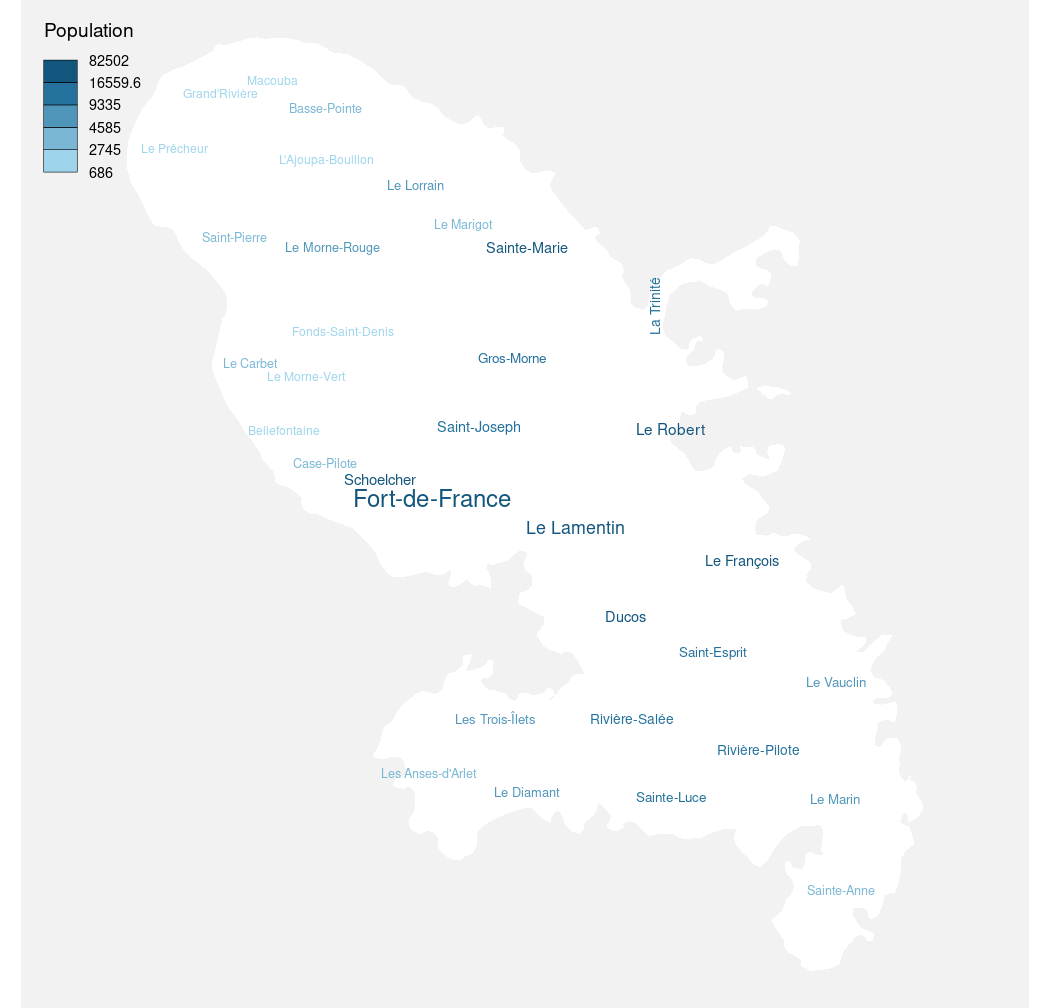Plot a word cloud adjusted to an sf object.

wordcloudLayer(
x,
txt,
freq,
max.words = NULL,
cex.maxmin = c(1, 0.5),
rot.per = 0.1,
col = NULL,
fittopol = FALSE,
use.rank = FALSE,
breaks = NULL,
method = "quantile",
nclass = NULL
)

## Arguments

x an sf object, a simple feature collection (POLYGON or MULTIPOLYGON). labels variable. frequencies of txt. Maximum number of words to be plotted. least frequent terms dropped integer (for same size in all txt) or vector of length 2 indicating the range of the size of the words. proportion words with 90 degree rotation color or vector of colors words from least to most frequent logical. If true would override rot.per for some elements of x logical. If true rank of frequencies is used instead of real frequencies. whether to add the layer to an existing plot (TRUE) or not (FALSE) additional arguments for adjusting the colors of txt, see choroLayer.

Ian Fellows (2018). wordcloud: Word Clouds.

R package version 2.6. https://CRAN.R-project.org/package=wordcloud

## Author

dieghernan, https://github.com/dieghernan/

## Examples

library(sf)
mtq <- st_read(system.file("gpkg/mtq.gpkg", package = "cartography"))
#> Reading layer mtq' from data source
#>   /tmp/RtmppBiN3A/temp_libpath43d97684eada/cartography/gpkg/mtq.gpkg'
#>   using driver GPKG'
#> Simple feature collection with 34 features and 7 fields
#> Geometry type: MULTIPOLYGON
#> Dimension:     XY
#> Bounding box:  xmin: 690574 ymin: 1592536 xmax: 735940.2 ymax: 1645660
#> Projected CRS: WGS 84 / UTM zone 20Npar(mar=c(0,0,0,0))
plot(st_geometry(mtq),
col = "white",
bg = "grey95",
border = NA)
wordcloudLayer(
x = mtq,
txt = "LIBGEO",
freq = "POP",`